×#### Thank you for registering.

One of our academic counsellors will contact you within 1 working day.

Click to Chat

1800-1023-196

+91-120-4616500

CART 0

• 0

MY CART (5)

Use Coupon: CART20 and get 20% off on all online Study Material

ITEM
DETAILS
MRP
DISCOUNT
FINAL PRICE
Total Price: Rs.

There are no items in this cart.
Continue Shopping• Complete JEE Main/Advanced Course and Test Series
• OFFERED PRICE: Rs. 15,900
• View Details

```Chapter 9: Trigonometric Ratios of Multiple and Sub Multiple Angles – Exercise 9.1

Trigonometric Ratios of Multiple and Sub Multiple Angles – Exercise 9.1 – Q.1

We have,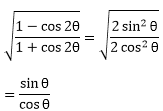= tan θ = RHS

Trigonometric Ratios of Multiple and Sub Multiple Angles – Exercise 9.1 – Q.2

LHS,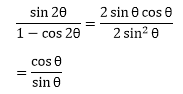= cot θ = RHS

Trigonometric Ratios of Multiple and Sub Multiple Angles – Exercise 9.1 – Q.3

LHS,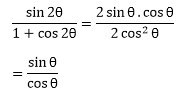= tan θ = RHS

Trigonometric Ratios of Multiple and Sub Multiple Angles – Exercise 9.1 – Q.4

LHS,= 2 cos θ =  RHS

Trigonometric Ratios of Multiple and Sub Multiple Angles – Exercise 9.1 – Q.5

LHS,= tan θ = RHS

Trigonometric Ratios of Multiple and Sub Multiple Angles – Exercise 9.1 – Q.6

LHS,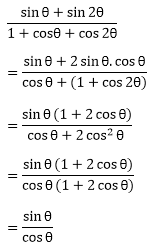= tan θ = RHS

Trigonometric Ratios of Multiple and Sub Multiple Angles – Exercise 9.1 – Q.7

LHS,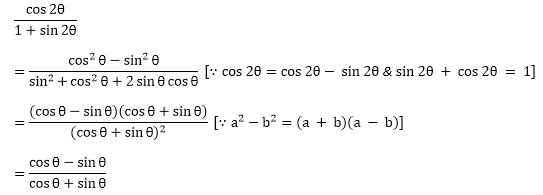Dividing numerator and denomenator by cos θ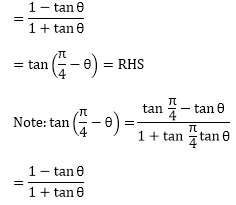Trigonometric Ratios of Multiple and Sub Multiple Angles – Exercise 9.1 – Q.8Dividing numerator and denominator by cos θ/2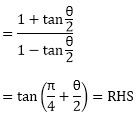Trigonometric Ratios of Multiple and Sub Multiple Angles – Exercise 9.1 – Q.9

LHS,= 2

= RHS

Trigonometric Ratios of Multiple and Sub Multiple Angles – Exercise 9.1 – Q.10

LHS,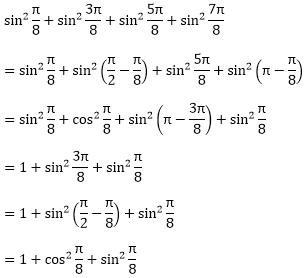= 1 + 1

= 2

= RHS

Trigonometric Ratios of Multiple and Sub Multiple Angles – Exercise 9.1 – Q.11

LHS,

(cos λ + cos β)2 + (sin λ + sin β)2

= cos2 λ + cos2 β + 2 cos λ cos β + sin2 λ + sin2 β + 2 sin λ sin β

= (cos2 λ + sin2 λ) + (cos2 β + sin2 β) + 2(cos λ cos β + sin λ sin β)

= 1 + 1 + 2cos (λ - β)

= 2 + 2 cos (λ - β)

= 2(1 + cos (λ - β)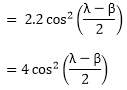= RHS

Trigonometric Ratios of Multiple and Sub Multiple Angles – Exercise 9.1 – Q.12

LHS,= RHS
```### Course Features

• 728 Video Lectures
• Revision Notes
• Previous Year Papers
• Mind Map
• Study Planner
• NCERT Solutions
• Discussion Forum
• Test paper with Video Solution